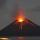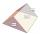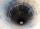# Volume + area of the shape - math problems

#### Number of problems found: 145

• Square prismCalculate the volume of a four-sided prism 2 dm high, and the base is a trapezoid with bases 12 cm, 6 cm, the height of 4 cm, and 5 cm long arms.
• Base of houseCalculate the volume of the bases of a square house. If the base depth is 1.2 m, the width is 40 cm, and their outer circumference is 40.7 m.
• VolcanoThe crater of a volcano is approximately in the shape of a cone of a base 3.1416 square miles. The crater's depth is 1500 ft. How many cubic yards of earth would be required to fill this cavity?
• A regularA regular triangular prism with a base edge of 20 dm and a height of 30 dm is drawn. Find the volume of the prism and the area of the shell.
• The wellThe well has the shape of a cylinder with a diameter of 2 m. From ground level to water level are 4 meters and the depth of water in the well is 6 m. How many m3 of soil did they dig when digging a well? How many liters of water is in the well?
• Annual rainfallThe average annual rainfall is 686 mm. How many liters will fall on the 1-hectare field?Calculate the surface area and volume of a regular quadrangular pyramid: sides of bases (bottom, top): a1 = 18 cm, a2 = 6cm angle α = 60 ° (Angle α is the angle between the sidewall and the base plane.) S =? , V =?
• Cylinder horizontallyThe cylinder with a diameter of 3 m and a height/length of 15 m is laid horizontally. Water is poured into it, reaching a height of 60 cm below the axis of the cylinder. How many hectoliters of water is in the cylinder?
• ContainerThe container has a cylindrical shape the base diameter 0.8 m and the area of the base is equal to the area of the wall. How many liters of water can we pour into the container?
• Rectangle 35Find the rectangle area when the diagonal is equal to 30 cms and the width is double the length.
• Triangular prismCalculate the volume and surface of the triangular prism ABCDEF with base of a isosceles triangle. Base's height is 16 cm, leg 10 cm, base height vc = 6 cm. The prism height is 9 cm.
• Children poolThe bottom of the children's pool is a regular hexagon with a = 60 cm side. The distance of opposing sides is 104 cm, the height of the pool is 45 cm. A) How many liters of water can fit into the pool? B) The pool is made of a double layer of plastic film
• Hexagonal prismThe base of the prism is a regular hexagon consisting of six triangles with side a = 12 cm and height va = 10.4 cm. The prism height is 5 cm. Find the volume and surface of the prism.
• Juice boxThe juice box has a volume of 200ml with its base is an isosceles triangle with sides a = 4,5cm and a height of 3,4cm. How tall is the box?The quadrangular prism has a volume of 648 cm3. Trapezoid which is its base has the dimensions bases: a = 10 cm, c = 5 and height v = 6 cm. What is the height of the prism?
• Square prismCalculate the volume of a square prism of high 2 dm wherein the base is: rectangle with sides 17 cm and 1.3 dm
• Cuboid and eq2Calculate the volume of cuboid with square base and height 6 cm if the surface area is 48 cm2.
• Chocolate rollThe cube of 5 cm chocolate roll weighs 30 g. How many calories will contain the same chocolate roller of a prism shape with a length of 0.5 m whose cross-section is an isosceles trapezoid with bases 25 and 13 cm and legs 10 cm? You know that 100 g of this
• Cube 5The content area of one cube wall is 32 square centimeters. Determine the length of its edges, its surface and volume.
• Tetrahedral prism - rhomboid baseCalculate the area and volume tetrahedral prism that has base rhomboid shape and its dimensions are: a = 12 cm, b = 70 mm, v_a = 6 cm, v_h = 1 dm.

Do you have an exciting math question or word problem that you can't solve? Ask a question or post a math problem, and we can try to solve it.

We will send a solution to your e-mail address. Solved examples are also published here. Please enter the e-mail correctly and check whether you don't have a full mailbox.

Tip: Our volume units converter will help you with the conversion of volume units. Volume - math problems. Examples of area of plane shapes.